• 对无偏估计值求方差
千次阅读
2019-08-01 10:57:56

今天的博客其实是对一个一直疑惑的数学知识点的讲解，毕竟机器学习还是运用到了大量的数学知识，所以把一些涉及到的数学概念搞清楚还是十分必要的。

方差其实这个概念相信大家都知道，就是 D ( x ) = 1 n ∑ i = 1 n ( x i − E ( x i ) ) 2 D(x)=\frac{1}{n}\sum_{i=1}^n(x_i-E(x_i))^2 ，其实就是每个样本值和均值均方差的平均值。但是可能会有人看过，在一些机器学习用到的场景中(比方说PCA)公式其实是 D ( x ) = 1 n − 1 ∑ i = 1 n ( x i − E ( x i ) ) 2 D(x)=\frac{1}{n-1}\sum_{i=1}^n(x_i-E(x_i))^2 ，虽然和之前的看上去非常像，但是会发现最后公式除的不是 n n 而是 n − 1 n-1 （原来我一直以为是书上印错成了 n − 1 n-1 ），这个微小的区别背后涉及到了很深刻的数学知识。

这里面包含了一个数学概念叫做“无偏估计”，“无偏估计”是一个什么概念呢？？
首先给一个严格数学上的定义即：对于随机变量 θ \theta 的估计是 θ ∗ \theta^* ，如果 E ( θ ) = E ( θ ∗ ) E(\theta)=E(\theta^*) ，那么就说参数 θ ∗ \theta^* 是参数 θ \theta 的无偏估计。
好像看上去有点不知道在说什么，这个其实就是我们一般在计算数据方差、均值等统计指标的时候，往往由于数据量过大无法计算全部样本，只能采用抽样统计的方式进行估计，比方说我想知道学校中男生的平均身高，很显然是没法通过全量样本来得到最终的目标值，只能按照某种方式进行采样，如每个班随机挑选5个人，然后计算所挑出样本的平均身高，最后近似得到全校男生的平均身高。你会发现在这个过程中，我们能计算统计的对象永远只能是筛选出的样本，而所谓无偏估计就是说筛选出样本的统计指标和全量样本的统计指标是一致的。

让我们再次回到这次要说的方差的例子， D ( x ) = 1 n ∑ i = 1 n ( x i − E ( x i ) ) 2 D(x)=\frac{1}{n}\sum_{i=1}^n(x_i-E(x_i))^2 计算的是样本的方差，而 D ( x ) = 1 n − 1 ∑ i = 1 n ( x i − E ( x i ) ) 2 D(x)=\frac{1}{n-1}\sum_{i=1}^n(x_i-E(x_i))^2 计算的是用样本来无偏估计得到的全量数据的真实方差，为什么前面乘的是 1 n − 1 \frac{1}{n-1} 而不是 1 n − 2 \frac{1}{n-2} 1 n − 3 \frac{1}{n-3} 呢？
这其实涉及到严格的数学证明，证明如下所示：
假设样本集方差为 S 2 S^2 ，全量数据真实方差为 σ 2 \sigma^2 ，样本集的均值为 x ∗ x^* ，全量数据真实均值为 μ \mu
S 2 S^2 = 1 n ∑ i = 1 n ( x i − x ∗ ) 2 \frac{1}{n}\sum_{i=1}^n(x_i-x^*)^2
= 1 n ∑ i = 1 n ( x i − μ + μ − x ∗ ) 2 =\frac{1}{n}\sum_{i=1}^n(x_i-\mu+\mu-x^*)^2
= 1 n ∑ i = 1 n [ ( x i − μ ) 2 + 2 ( x i − μ ) ( μ − x ∗ ) + ( μ − x ∗ ) 2 ] =\frac{1}{n}\sum_{i=1}^n[(x_i-\mu)^2+2(x_i-\mu)(\mu-x^*)+(\mu-x^*)^2]
= 1 n ∑ i = 1 n ( x i − μ ) 2 + 2 n ∑ i = 1 n ( x i − μ ) ( μ − x ∗ ) + 1 n ∑ i = 1 n ( μ − x ∗ ) 2 =\frac{1}{n}\sum_{i=1}^n(x_i-\mu)^2+\frac{2}{n}\sum_{i=1}^n(x_i-\mu)(\mu-x^*)+\frac{1}{n}\sum_{i=1}^n(\mu-x^*)^2
= 1 n ∑ i = 1 n ( x i − μ ) 2 + 2 n ( μ − x ∗ ) ∑ i = 1 n ( x i − μ ) + 1 n ∑ i = 1 n ( μ − x ∗ ) 2 =\frac{1}{n}\sum_{i=1}^n(x_i-\mu)^2+\frac{2}{n}(\mu-x^*)\sum_{i=1}^n(x_i-\mu)+\frac{1}{n}\sum_{i=1}^n(\mu-x^*)^2
= 1 n ∑ i = 1 n ( x i − μ ) 2 + 2 n ( μ − x ∗ ) ∑ i = 1 n ( x ∗ − μ ) + 1 n ∑ i = 1 n ( μ − x ∗ ) 2 =\frac{1}{n}\sum_{i=1}^n(x_i-\mu)^2+\frac{2}{n}(\mu-x^*)\sum_{i=1}^n(x^*-\mu)+\frac{1}{n}\sum_{i=1}^n(\mu-x^*)^2
= 1 n ∑ i = 1 n ( x i − μ ) 2 − 2 n ( μ − x ∗ ) ∑ i = 1 n ( μ − x ∗ ) + 1 n ∑ i = 1 n ( μ − x ∗ ) 2 =\frac{1}{n}\sum_{i=1}^n(x_i-\mu)^2-\frac{2}{n}(\mu-x^*)\sum_{i=1}^n(\mu-x^*)+\frac{1}{n}\sum_{i=1}^n(\mu-x^*)^2
= 1 n ∑ i = 1 n ( x i − μ ) 2 − 1 n ( μ − x ∗ ) ∑ i = 1 n ( μ − x ∗ ) =\frac{1}{n}\sum_{i=1}^n(x_i-\mu)^2-\frac{1}{n}(\mu-x^*)\sum_{i=1}^n(\mu-x^*)
= σ 2 − D ( x ∗ ) =\sigma^2-D(x^*)

其中 D ( x ∗ ) = D ( 1 n ∑ i = 1 n x i ) = 1 n 2 D ( ∑ i = 1 n x i ) D(x^*)=D(\frac{1}{n}\sum_{i=1}^nx_i)=\frac{1}{n^2}D(\sum_{i=1}^nx_i)
由于样本都是独立同分布的故有 D ( x i + x j ) = D ( x i ) + D ( x j ) D(x_i+x_j)=D(x_i)+D(x_j)
则上述有 D ( x ∗ ) = 1 n 2 D ( ∑ i = 1 n x i ) = 1 n 2 ∑ i = 1 n D ( x i ) = 1 n 2 n D ( x i ) = 1 n σ 2 D(x^*)=\frac{1}{n^2}D(\sum_{i=1}^nx_i)=\frac{1}{n^2}\sum_{i=1}^nD(x_i)=\frac{1}{n^2}nD(x_i)=\frac{1}{n}\sigma^2
将其带入上式则有
S 2 = σ 2 − D ( x ∗ ) = σ 2 − 1 n σ 2 = n − 1 n σ 2 S^2=\sigma^2-D(x^*)=\sigma^2-\frac{1}{n}\sigma^2=\frac{n-1}{n}\sigma^2
即有 σ 2 = n S 2 n − 1 \sigma^2=\frac{nS^2}{n-1}
可以发现真正全量数据无偏估计的方差其实和样本数据的方差还是有一定区别的，从公式我们也可以发现最后为什么前面的系数是 1 n − 1 \frac{1}{n-1} 而不是其他的值，这其实是涉及到了深刻的数学知识，绝不是人拍脑袋的一个值。

更多相关内容
• 1）基本概念 我们先从最基本的一些概念入手。...应该把样本和总体样本一样进行抽象化理解，因此样本也存在期望和方差。 这里有一个重要的假设，就是随机选取的样本与总体样本同分布，它的意思...

### 1）基本概念

我们先从最基本的一些概念入手。

如下图，脑子里要浮现出总体样本，还有一系列随机选取的样本。只要是样本，脑子里就要浮现出它的集合属性，它不是单个个体，而是一堆随机个体集合。样本是总体样本中随机抽取一系列个体组成的集合，它是总体样本的一部分。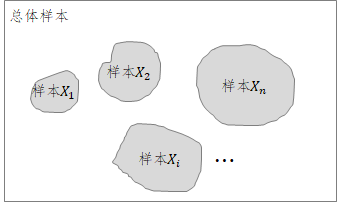应该把样本和总体样本一样进行抽象化理解，因此样本也存在期望和方差

这里有一个重要的假设，就是随机选取的样本与总体样本同分布，它的意思就是说他们的统计特性是完全一样的，即他们的期望值一样，他们的方差值也是一样的：

另外，由于每个样本的选取是随机的，因此可以假设不相关(意味着协方差为0，即)，根据方差性质就有:

另外，还需要知道方差另外一个性质：

为常数。

还有一个，别忘了方差的基本公式：

以上的公式都很容易百度得到，也非常容易理解。这里不赘述。

### 2）无偏估计

接下来，我们来理解下什么叫无偏估计。

定义：设统计量是总体中未知参数的估计量，若，则称无偏估计量；否则称为有偏估计量。

上面这个定义的意思就是说如果你拿到了一堆样本观测值，然后想通过这一堆观测值去估计某个统计量，一般就是想估计总体的期望或方差，如果你选择的方法所估计出来的统计量的期望值与总体样本的统计量相等，那么我们称这种方法下的估计量是无偏估计，否则，就称这种方法下的估计量为有偏估计量。

按照这么理解，那么有偏无偏是针对你选择估计的方法所说的，它并不是针对具体某一次估计出来的估计量结果。如果方法不对，即使你恰好在某一次计算出来一个值和总体样本统计量值相同，也并不代表你选的这个方法是无偏的。为什么呢？这是因为单次值是和你选取的样本相关的，每次样本（更加严格的意义是某次样本快照）的值变化了，那么每次的值就有可能跟着变化，你就需对这么多求期望值来判断的可信程度，如果一直重复这个试验，然后它的期望值与总体样本的统计量一样，那么称按照这种方法估计出来的统计量是无偏的。

来一点题外话：

但凡是想通过有限的信息去"估计"一个整体的"量"，这种情形下谈这个"估计"的方法“有偏”\“无偏”才有意义。一般来说，这种情形下，这个被估计的"量"肯定是有碍于技术或者现实情况无法严格准确获取，比如因为成本过高这些"量"无法通过穷举或者其他办法获知。否则，如果被估计的"量"很容易获取，就不需要"估计"了，采用统计方法就可以了。

如果你只是要进行简单的"统计"就能获得你想要的"量"，那么没必要去关心所采用的方法是"有偏"还是“无偏"；尤其是当整体信息很容易获取的情况下谈"有偏"还是“无偏"就毫无意义。比如要谈某个班级的身高的平均值，直接将身高总数除以班级人数就可以了，因为根本没必要去"估计"，因为它仅仅是个"统计"问题；同样的，求一个班级的身高方差也不用任何纠结，求方差过程中除以班级人数就OK了，没有必要非常变态的研究是除以"班级总人数"还是"班级总人数-1"，你要是去纠结这个，那就是吃饱了撑的了。但是，假如学校有几万人，你要统计的是整个学校所有的人的平均身高，这个时候一个一个进行统计是不现实的，反而需要使用的"估计"的方法。你采用的方法是随便抓100个人过来，将这100人总的身高数值除以100，估计出来的平均值就可以假设认为是整个学校的身高平均值，因为，你是用部分样本估计了总体样本的一个”量“，所以这个是"估计"；此时，要是估计整个学校学生身高的方差，如果要想估计方法"无偏", 那就不是除以100了，而是除以99。当然，如果你是一位粗人，无所谓啥"有偏"还是“无偏"的束缚，那么你直接除以100也不会遭到嘲笑的，具体原因得继续往下看。总之，无法通过整体直接"统计"获得你想要的"量"时，你只能通过"部分样本"来做"整体样本""量"的估计时，谈估计方法的"有偏"还是"无偏"才是有意义的。

### 3）样本均值的无偏估计

接下来探讨一下下面的结论：

定理1：样本均值是总体样本均值的无偏估计。

注意：这里样本均值不是指某个样本的均值。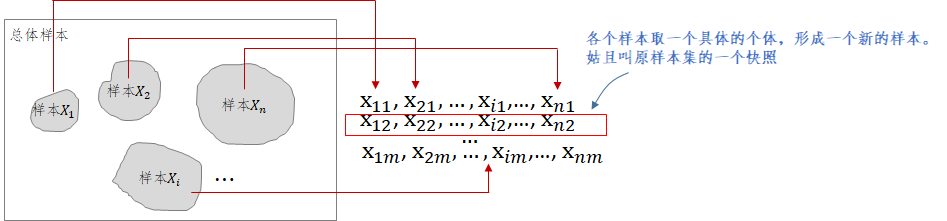这里需要看上面这张图，这里的均指的是特定某次样本集合的快照（上图红色框）,显然这个快照也是一个样本，只不过这个样本它的样本大小固定为n，这与抽象的样本不一样（一般我们想象抽象的样本，比如，是无限大的）。

明显，

第一个样本(快照)均值是长这样子的：

第二个样本(快照)均值是长这样子的：

....依此类推...

表示第次随机从从本获取一个个体。

试验一直进行下去，你就会有一些列估计出来的样本(快照)均值，实际上这也称为了一个样本，我们称为均值的样本，既然是样本，它就也有统计量。我们这里重点关注这个均值样本的期望。因为按照估计量的有偏无偏定义，如果，那么按照这个方法估计的均值就是无偏的。仔细思考，估计量有偏无偏它是针对你所选定的某个估计方法所形成的估计量样本空间来讨论的，讨论单次试验形成的估计量是没有太大意义的，只有针对形成的估计量样本空间才有意义。

下面验证上面的方法形成的估计是无偏的。

这么一来，就和教科书和网上的资料结果上都对上了，教科书上的公式在下面列出（符号用代替）：

有了前面的分析，上面的教科书公式就很好理解了，注意，里头的是原始样本，也是样本!!!  公式推导过程中，表示了原始的样本快照求和后再除以n形成的估计量样本，所以是可以对其再进行求期望的。

讨论完估计量样本的均值，我们别忘了，既然它是个样本，那么可以计算的方差（后面会用到）:

所以，样本（快照）均值的期望还是总体期望，但是，样本（快照)均值的方差却不是原来的方差了，它变成原来方差的1/n。这也容易理解，方差变小了是由于样本不是原来的样本了，现在的样本是均值化后的新样本，既然均值化了，那么比起原来的老样本，它的离散程度显然是应当变小的。

### 4）样本方差的无偏估计

定理2：样本方差是总体样本方差的无偏估计。

也就是需要证明下面的结论：

首先，脑子里要非常清楚，你截至目前，仅仅知道以下内容：

其中前面5个来自1），最后2个来自3)。

至于为什么是，而不是，需要看下面的证明。

那么为什么会导致这么个奇怪的结果，不是而是  ？

仔细看上面的公式，如果，那么就应该是了，但是残酷的事实是（除非本身就等于0），导致的罪魁祸首是。这就有告诉我们，虽然将方差缩小了n倍，但是仍然还有残存，除非本身就等于0，才会有，但这就意味着所有样本的个体处处等于

还有一种情况，如果你事先就知道，那么就是的无偏估计，这个时候就是了。

---------------------------------------------------------------------------------------------------------------------------------------------

有人还是问我为什么（总体均值）已知，就可以用作为总体方差的无偏估计，这个完全直接推导就可以证明。证明如下：

这个结论告诉我们，如果某个人很牛逼，他可以知道确切的总体样本均值，那么就可以用来估计总体样本方差，并且这个估计方法是保证你无偏的。

而上面的，请睁大眼睛看清楚，用的是。大部分的实际应用情况下，谁也不知道总体样本均值(请问你知道全球人均身高么？鬼知道，地球上没有一个人可以知道！我想即使是外星爸爸也不知道！)，但是我还是想在全球人都不知道的情况下去估计总体的身高方差，怎么办？现在有个办法，我们可以去抓一些人（部分样本）来做一个部分样本均值，那就用部分样本均值也就是来近似代表（上面的定理1告诉我们这种方法对于估计是无偏的），但是现在我想估计另外一个东东，那个东东叫总体样本方差。好了，我们可以也用代入来估计总体样本方差，并且如前面所分析的，这个估计方法针对是无偏的。（至于为啥是奇怪的，简单直接的原因是因为我不知道总体样本均值，因为如果你能够知道，我们就可以不需要用奇怪的，我们就可以用去估计总体样本方差）。

总之，是理论上的总体样本方差。是实际应用中采用的总体样本方差估计。

------------------------------------------------------------------------------------------------------------------------------------------------------------------

统计学中还有一个"自由度"的概念。为什么是除以n-1还可以从自由度角度进行解释，具体可以参看下面百度的解释：

自由度 （统计学的自由度）

展开全文• ## 方差的无偏估计

千次阅读 2020-05-21 21:52:30
方差无偏估计如何计算？ 我们常常被问到"方差无偏估计如何计算？和有偏估计的区别是什么？"，心想"哎呀，又忘了"。本篇回归问题本质，带你理解这些名词背后解决的实际问题（通过总结回顾，无意中解决了一年...

方差的无偏估计如何计算？

参考：
https://www.zhihu.com/question/20099757


我们常常被问到"方差的无偏估计如何计算？和有偏估计的区别是什么？"，心想"哎呀，又忘了"。本篇回归问题本质，带你理解这些名词背后解决的实际问题（通过总结回顾，无意中解决了一年以来萦绕脑海的遗留问题，开森~~）。

### 一、基本概念

解题第一步是理解题意，通过示例首先搞清楚以下几个概念。

假如你想调研所在大学女生的身高，你站在厕所门口（女生一般爱上厕所^~^），随机去问n个女生（独立同分布），最后通过哪些数值来反映身高呢？一般我们都会使用均值。

但如果在调研的时候，你发现有的女生特别高（猜测是校篮球队的），该样本并不能真实反映女生普遍身高，这就导致采集的样本存在异常数据，那么你可以通过方差来度量身高的差异。

由于学校的全体女生身高的均值µ 和方差σ未知，这里通过采样计算得到的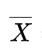和 S2，都只是对已知分布中的未知参数的一个估计，这就是估计量。在估计时用到的样本均值和样本方差是用来描述数据特征的，被叫做是统计量。

上面示例提到以下概念，严格定义如下：

• 期望

是指随机事件中随机变量和它出现概率的乘积的总和，反映了随机变量平均取值的大小，又称"均值"。

E(X) = Σip(xi)xi

• 方差

是用来度量随机变量和其均值之间的偏离程度，方差越小，偏离程度越小。

D(X) = E([X-E(X)]2)

• 统计量

已知样本集，由样本值计算的函数，被称为统计量，不含未知参数。比如样本平均值，样本方差，样本标准差等。

• 估计量

设总体样本的分布函数已知，参数未知。已知样本集，需要构造适当的统计量来估计未知参数的近似值，这被称为估计量。

### 二、那么问题来了

以上示例中两个指标的计算方式如下：

样本均值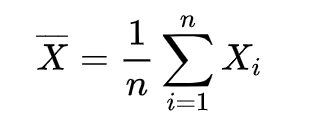样本方差为什么方差的计算分母是n-1，而不是n ？

实际上示例中的统计量是对未知参数的估计，而估计量的选择是有评价标准的，以下是三种常见的评价指标，这里只考察估计量的无偏性。

### 三、估计量的评价标准

#### 1. 无偏性

若估计量的数学期望存在，且期望等于未知参数，则称该估计量为参数的无偏估计量。

估计量的无偏性是指对于某些样本值来说，得到的估计量和真值相比，有的偏大，有的偏小，但就其平均而言，偏差为0。估计量的期望和真值相差被称为系统误差，无偏估计实际上是指无系统误差。

#### 2. 有效性

设有两个无偏估计量，都是真值的估计，其中方差小的估计量较方差大的更有效。

估计量的有效性，是希望无偏估计量取值偏离真值的程度越小越好，所以以方差小的估计量更好。

#### 3. 相合性

随着样本数无限增加，估计量依概率收敛于真值，则被称为相合估计量。

以上两个标准都是以样本数固定为前提，我们希望随着样本的增加，估计量的值趋近于参数的真值。

### 四、方差的无偏性

由以上无偏性标准的定义可知，方差的无偏估计需要估计量的均值等于方差真值，当分母是n时，如下公式可见

#### 1. 公式推导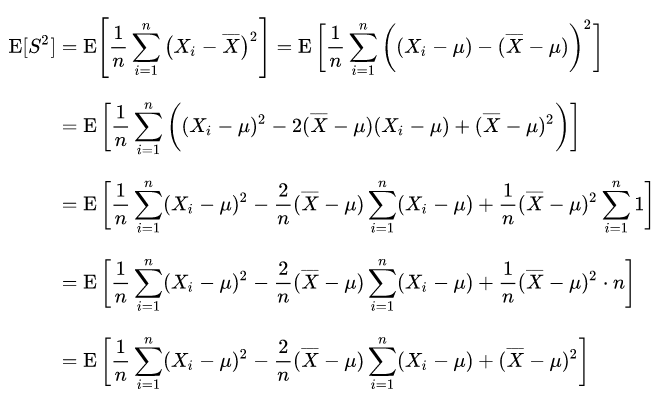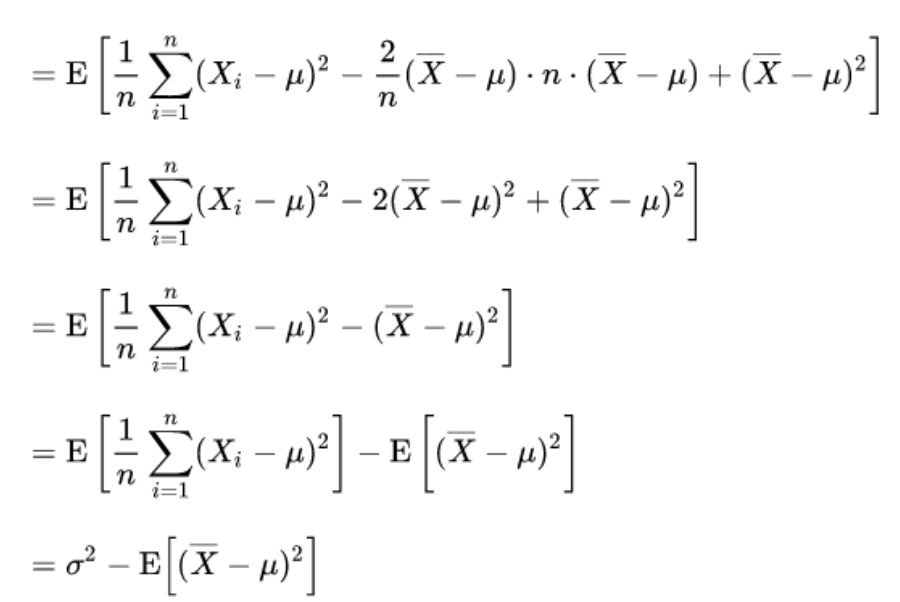所以，只有样本均值等于真值均值时，样本方差的均值才等于真值方差。由于样本的随机性，样本均值取值不一定，所以分母为n的估计量均值 <= 真值方差，为有偏估计。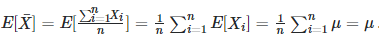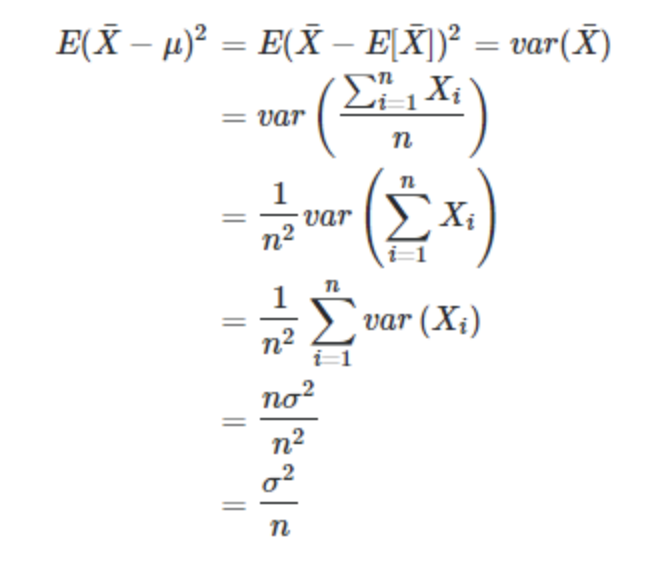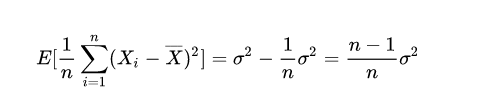即下式是对方差的无偏估计#### 2.通俗理解（自由度）

计算估计量的样本需要独立同分布，由于分布参数未知，使用样本均值来计算样本方差时，样本均值是由各样本计算而来。假设样本容量为n，已知n-1个样本值，可由样本均值推断出最后一个样本取值，破坏了样本独立性，故该样本集的自由度为n-1，所以计算样本方差时样本数应该减去1。

参考：

https://www.zhihu.com/question/20099757

《概率论与数理统计》

展开全文• 样本方差无偏估计样本方差问题背景S2S^2S2的近似作用为什么使用Xˉ\bar XXˉ替代后，分母是1n\frac{1}{n}n1​？无偏估计无偏性有效性一致性小结 参考文章：如何理解无偏估计量？ by 马同学 样本方差 问题背景 首先...

参考文章：如何理解无偏估计量？ by 马同学

## 样本方差

### 问题背景

首先，对于随机变量 X X 的期望为 μ \mu ，其方差为 σ 2 \sigma^2

如果已知随机变量X的期望为 μ \mu ，那么可以如下计算方差 σ 2 \sigma^2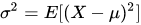↑Case 1: 分布和期望均已知

不过，对于上式，首先需要知道 X X 的具体分布。因而我们实践中常常采样之后，常采用如下方式近似估计方差：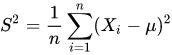↑Case 2: 分布未知，期望已知

然而，实际中我们对期望也是未知的（Case 3），只能通过样本得到均值 X ˉ \bar X ，那么我们可以通过样本方差公式计算 S 2 S^2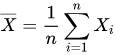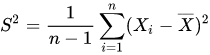### S 2 S^2 的近似作用

首先，我们从Case 2开始分析。

对于某一 σ 2 = 1. 4 2 = 1.96 \sigma^2=1.4^2=1.96 （未知），期望 μ \mu 已知的正态分布，随机采样，并通过下图公式估计 σ 2 \sigma^2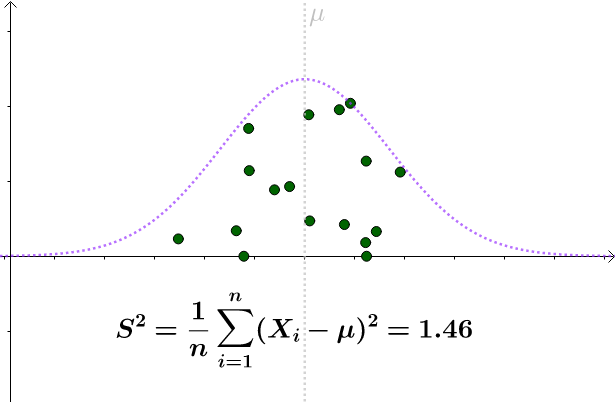上图只是随机采样的一种情况。多采样几次， S 2 S^2 会围绕 σ 2 \sigma^2 上下波动。因此，这样估计在可接受范围内。很容易算出：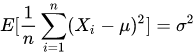同时，根据中心极限定理， S 2 S^2 采样的均值会服从已知期望平方 μ \mu 的分布。

这也就是所谓的无偏估计量。

### 为什么使用 X ˉ \bar X 替代后，分母是 1 n \frac{1}{n} ？

对于更常见的Case 3，未知更多，只能得出平均值 X ˉ \bar X

对于某次采样而言，当 X ˉ = μ \bar X = \mu 时，下式取得最小值：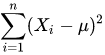但是，不同于Case 2，对于Case 3 下的每次采样，很难避免样本均值偏离期望这一情况的发生。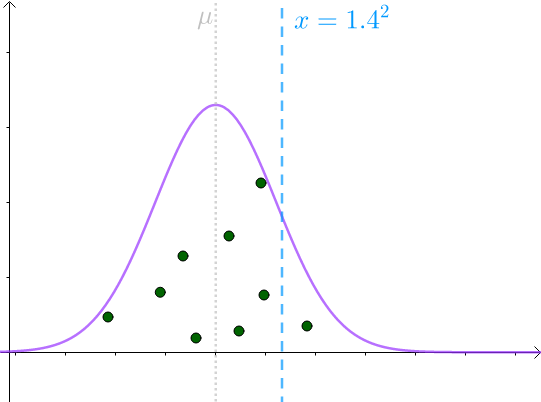因此，如果我们用 X ˉ \bar X 近似 μ \mu ，有：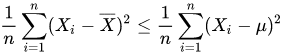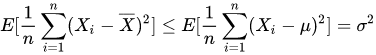所以，如果用下面这个式子来估计：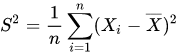S 2 S^2 采样的均值会服从一个偏离真实期望平方 μ \mu 的分布，且倾向于低估 μ \mu

下面援引计算偏移量的推导：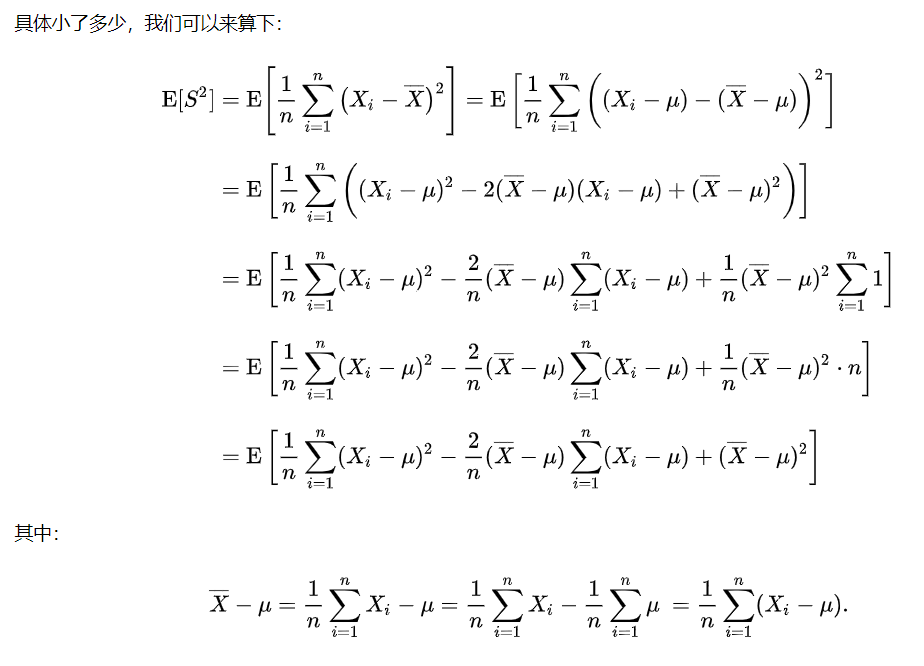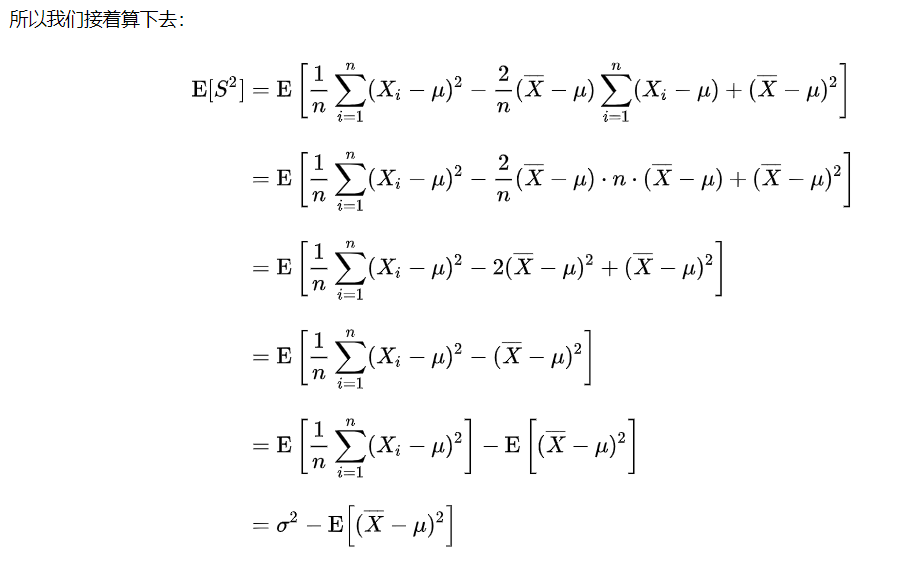因为
E [ ( X ˉ − μ ) ] 2 = [ 1 n ∑ ( X i − μ ) ] 2 = 1 n [ 1 n ∑ ( X i − μ ) 2 ] = 1 n σ 2 E[(\bar X-\mu)]^2=[\frac{1}{n}\sum(X_i-\mu)]^2=\frac{1}{n}[\frac{1}{n}\sum(X_i-\mu)^2]=\frac{1}{n}\sigma^2
这就是偏移量。

所以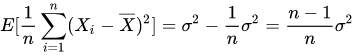进行一下调整，得到无偏估计下的样本方差：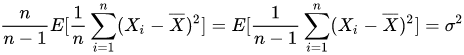## 无偏估计

### 无偏性

例如平均值，就是对样本的一个不错的估计，因为它属于无偏估计。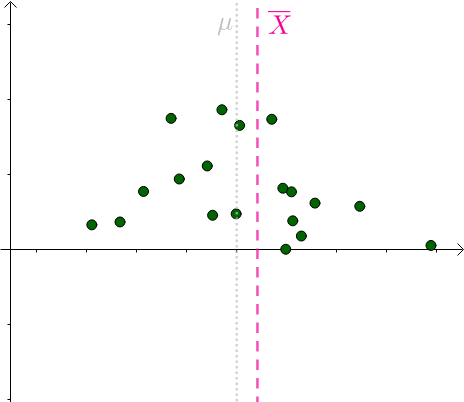可以发现，不同采样得到的 X ˉ \bar X 是围绕 μ \mu 左右波动的。

而前面提到的样本方差，如果用下式去估计方差：则会产生偏差，属于有偏估计。这种偏差像是瞄准镜一直歪斜，是系统性的。

### 有效性

简单的说，就是估计量越靠近目标，效果越好。这个“靠近”也可以用方差来衡量（注意这里的衡量和上面的样本方差估计是两回事）。

有效估计和无偏估计是不相关的：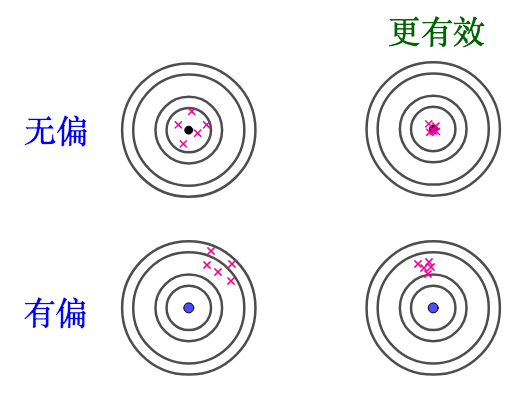假设有10个样本，估计样本均值，下面两个都是无偏估计量，只是后者可能更有效：若只优先考量有效性，无偏和有偏估计没有优劣之分：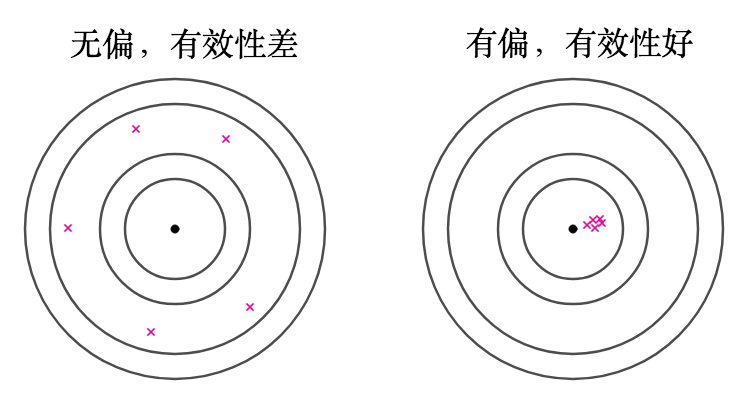### 一致性

在前面介绍的样本方差的例子中，如果采用那么对于偏差 1 n σ 2 \frac{1}{n}\sigma^2 ，可以看到，随着采样个数n的增加，这个偏差会越来越小。那么这个估计就是“一致”的。如果样本数够多，其实这种有偏但是一致的估计量也是可以采用的。

## 小结

判断一个估计量“好坏”，可以考虑以下几个方面：

• 无偏性
• 有效性
• 一致性
展开全文• 偏估计 and 无偏估计 方差分母 n-1 - 详细证明 均值 - 无偏估计 方差 - 有偏估计 方差 - 转换成无偏估计有偏估计
• - 确定好的估计量 - 建立数据的数学模型：一般由于数据固有的随机性，则选择它们的PDF来描述它，...- 最佳估计量的选择：估计量性能的评估（无偏性、有效性以及一致性）。常用的方法：期望验证无偏；CRLB（Cramer-R...
• 下面首先 β \beta β的估计值 β ^ \hat{\beta} β^​，并证明它是 β \beta β的无偏估计，先不考虑（1）式中的误差项，并将有所的样本带入上市，我们可得： Y = X T β Y = X^T\beta Y=XTβ − ( 2 ) \ \ \ \ ...机器学习 人工智能
• 无偏估计常被应用于测验分数统计中，以下将呈现证明样本方差不是总体方差无偏估计的两种方法。
• 是关于方差无偏估计，那么为什么一个是/(n-1），为什么一个是/n呢 首先我们清楚几个公式， D(x)= E(x)= 有一个重要的假设，就是随机选取的样本与总体样本同分布，它的意思就是说他们的统计特性是完全一样的，...
• 未决赔款准备金的一致最小方差无偏估计，张丽亚，吴黎军，使用当前保险实务中常用的未决赔款准备金计提方法，我们仅仅可以得到未决赔款准备金的一个估计值，而方差却没有研究。本文基
• 我们常常被问到"方差无偏估计如何计算？和有偏估计的区别是什么？"，心想"哎呀，又忘了"。本篇回归问题本质，带你理解这些名词背后解决的实际问题（通过总结回顾，无意中解决了一年以来萦绕脑海的遗留问题，开森~~...
• 综合资料一二三，分析（有偏和无偏估计量）方差。 资料一： 资料二： 资料三： 资料四： 分析： 此处包含方差的一个公式，方差 = 平方的期望 - 期望的平方 ...
• 什么叫无偏估计？为什么方差的定义里写的是1/n，但又说无偏估计是1/(n-1)？本文本着一切都从定义开始的原则，推导无偏估计方差
• （一）高斯分布  高斯分布又称正态分布，是大自然中最常见的一种数据分布方式，在机器学习的各类算法中，经常要遇到随机数的生成，我们通常采用高斯分布来进行随机数的...（二）无偏估计  现实生活中，通常要面...高斯分布
• - 回顾 ...前面一直在讲述估计量的有效性（CRLB，线性模型），而没有提到假如估计量的方差没有达到CRLB，即是有效估计量不存在，但能够出MVU估计量（假定存在）仍然是一个重要的事（可参考文章中的图片...
• 文章目录1、无偏估计量2、最小方差准则3、最小方差无偏估计的存在性4、最小方差无偏估计量5、扩展到矢量参数 1、无偏估计量   未知参数进行估计，得到估计量。而所谓无偏估计，是指估计量的均值，等于未知参数...
• 最小方差无偏估计 2.1 无偏估计量 ​ 无偏估计意味着估计量的平均为未知参数的真值。如果 E[θ^]=θ,a<θ<b E[\hat{\theta}]=\theta, a<\theta<b E[θ^]=θ,a<θ<b 说明估计量θ^\hat{\theta}θ...
• 被解释变量的估计值与观测值的残差 e=Y−Xβ^=Xβ^+μ−X(X′X)−1X′(Xβ^+μ)=μ−X(X′X)−1μ=[I−X(X′X)−1X′]μ=Mμe=Y-X\hat\beta \\=X\hat\beta+\mu-X(X'X)^{-1}X'(X\hat \beta+\mu) \\=\mu-X(X'X)^{-1}\...
• 偏和有偏本质来讲，偏/无偏估计是指估算统计量的公式，无偏估计就是可以预见，多次采样计算的统计量(根据估算公式获得)是在真实左右两边。类似于正态分布的钟型图形。比如对于均值估计：mean = (1/n)Σxi一定...
• 均值 X‾=∑i=1nXin\overline X=\frac{\sum_{i=1}^{n}X_i}{n}X=n∑i=1n​Xi​​ ...方差 s=∑i=1n(Xi−X‾)2n−1s=\frac{\sum_{i=1}^{n}(X_i-\overline X)^2}{n-1}s=n−1∑i=1n​(Xi​−X)2​ 为什
• 来一点废话，帮助大家理解概率的精髓： 1） 只要谈估计，那就是告诉你一种方法，利用这个方法可以管中规豹似的获取某个统计量（这个统计量很可能限于人力物力无法真正获取，而...的无偏估计量。 其中是样本均值，...
• 虽然对无偏估计有所了解，但是还是有些问题：1)总体期望的无偏估计量是样本均值x-，总体方差的无偏估计是样本方差S^2,为什么样本方差需要除以n-1,而不是除以n;2)样本在总体中是怎样的抽样过程，是放回抽样，是随机...
• &nbsp; &nbsp; 设样本均值为，样本方差为，总体均值... 很多人可能都会有疑问，为什么要除以n-1，而不是n，但是翻阅资料，发现很多都是交代到，如果除以n，样本方差的估计不是无偏估计，比总体方差要小，...
• 样本方差无偏估计与（n-1）的由来 一、无偏估计 所谓总体参数估计量的偏性指的是，基于不同的样本，使用该估计量可算出多个估计值，但它们的平均值等于被估参数的真值。  在某些场合下，...
• 在之前的学习中，主要基于充分统计量给出点估计，并且注重于点估计无偏性与相合性。然而，仅有这两个性质是不足的，无偏性只能保证统计量的均值与待估参数一致，却无法控制统计量可能偏离待估参数的程度；相合性...
• 什么是无偏估计？？ 估计是用样本统计量（可以理解为随机抽样）来估计总体参数时的一种偏推断。 无偏估计的要求就是：估计出来的参数的数学期望等于被估计参数的真实值。 所以呢,可以看出：估计值也是一个变量，...
• 3.为什么样本方差会是有偏估计值呢？ 样本方差偏估计为 σ ^ m 2 = 1 m − 1 ∑ i = 1 m ( x i − μ ^ m ) \hat\sigma^2_{m}=\frac{1}{m-1}\sum^{m}_{i=1}{(x^{i}-\hat\mu_{m})} σ ^ m 2 ​ = m − 1 1 ​ ...
• 什么是无偏估计？？ 估计是用样本统计量（可以理解为随机抽样）来估计总体参数时的一种偏推断。 无偏估计的要求就是：估计出来的参数的数学期望等于被估计参数的真实值。 所以呢,可以看出：估计值也是一个变量，......